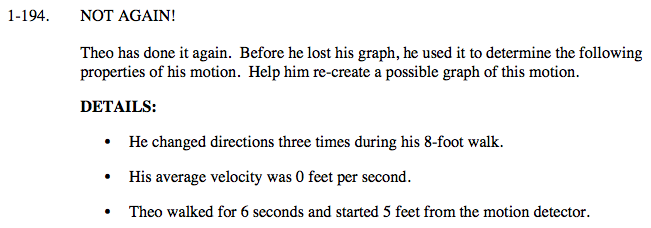### Home > CALC > Chapter 1 > Lesson 1.5.1 > Problem1-194

1-194.Clue 1: A change in direction means the slope goes from positive to negative or negative to positive. An 8-foot walk means that, when you add together all the distances traveled between each high and low point on the graph, the sum is 8.

Clue 2: An average velocity of 0 means that Theo should end exactly where he started.

Clue 3: If Theo walked for 6 seconds, then the domain of the graph is restricted to 0≤x≤6. If Theo started 5 feet from the motion detector, then the y-intercept is (0,5)